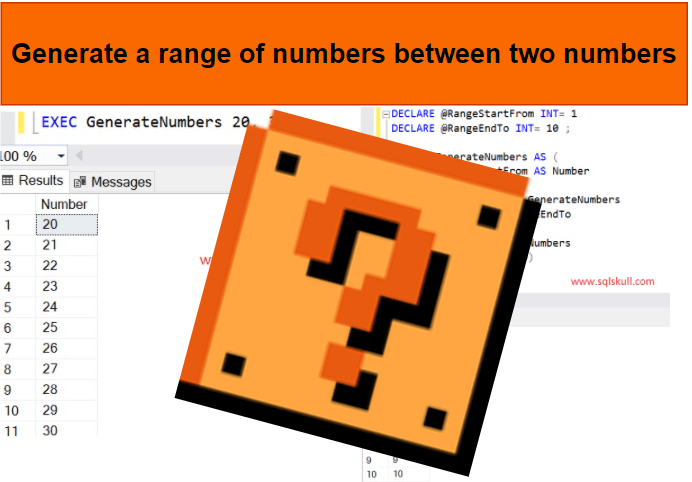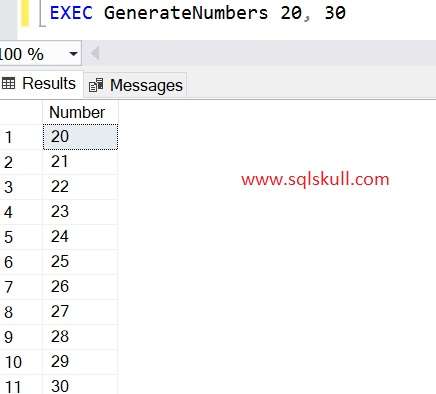Home » Generate a range of numbers between two numbers in SQL Server

# Generate a range of numbers between two numbers in SQL ServerSometimes, you need to show range of numbers or may be asked to generate a range of numbers between two numbers in SQL Server.

Well, it is pretty simple and can be done using different-different approaches, but one of the most common and easiest way of doing it is using a Recursive CTE.

Lets create a query that uses a recursive CTE and returns a range of numbers between two numbers.

Following SQL query returns the numbers between two specified numbers that is 1 and 10.

```DECLARE @RangeStartFrom INT= 1
DECLARE @RangeEndTo INT= 10 ;

; WITH GenerateNumbers AS (
SELECT @RangeStartFrom AS Number
UNION ALL
SELECT Number +1 FROM GenerateNumbers
WHERE Number +1<= @RangeEndTo
)
SELECT * FROM GenerateNumbers
option (MAXRECURSION 0)```

So, when you execute the above query, it will return the numbers from 1 to 10.

Lets execute the query and see the output.As you can see, it returns numbers starting from 1 to 10.

Also you can convert this query into stored procedure that will take two input parameters for range and returns the ranges of numbers based on those parameter values.

Lets create a stored procedure as shown below.

```CREATE PROC GenerateNumbers
@RangeStartFrom AS INT = 0,
@RangeEndTo AS INT =0
AS
BEGIN
; WITH GenerateNumbers AS (
SELECT @RangeStartFrom AS Number
UNION ALL
SELECT Number +1 FROM GenerateNumbers
WHERE Number +1<= @RangeEndTo
)
SELECT * FROM GenerateNumbers
option (MAXRECURSION 0)

END```

Lets execute the stored procedure, this time we we generate the range of numbers between 20 to 30.Recursive CTE

SQL Server Interview Question and Answers

SQL Server Basics Concepts1.